Anúncio

# B.sc(microbiology and biotechnology and biochemistry) ii inorganic chemistry unit 3.2 covalent bond

17 de Mar de 2015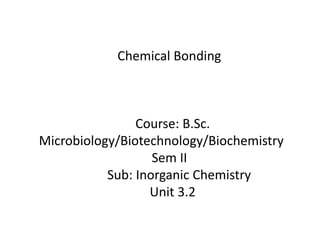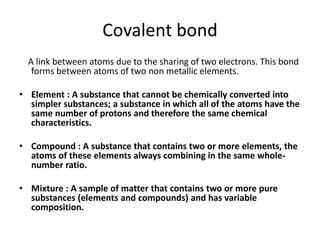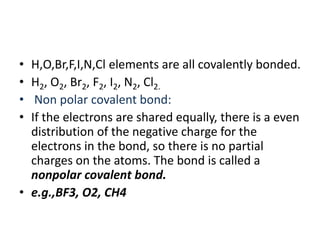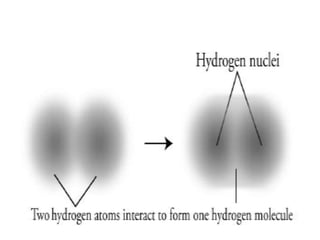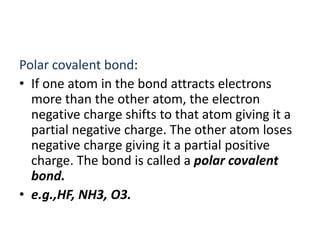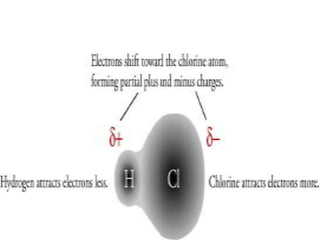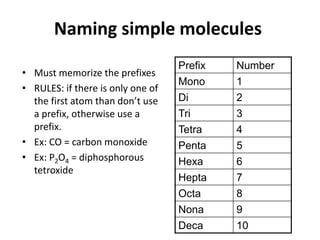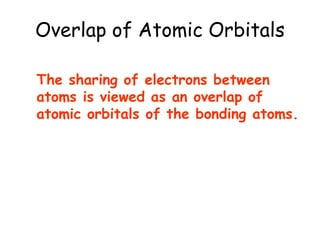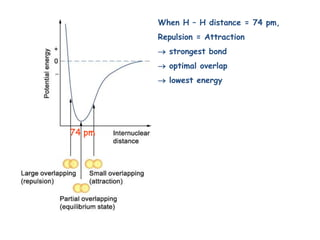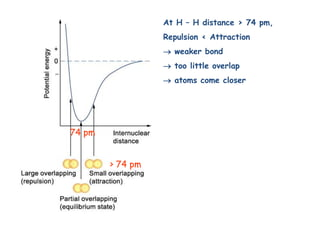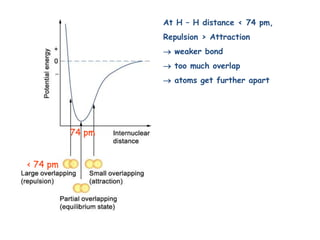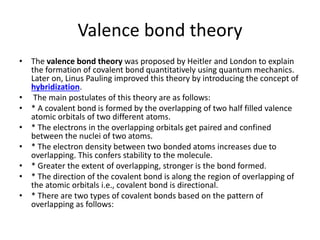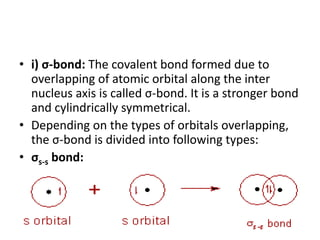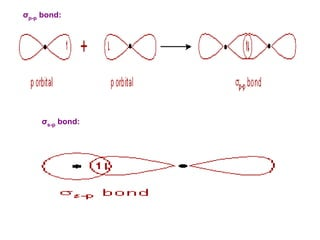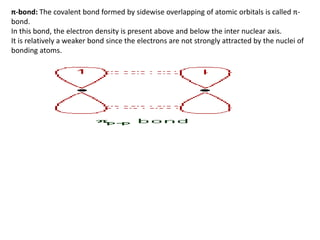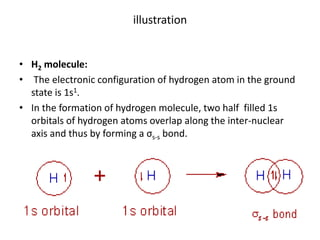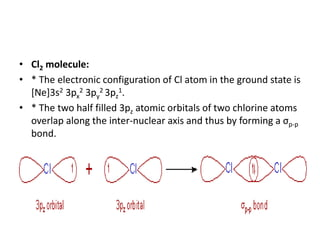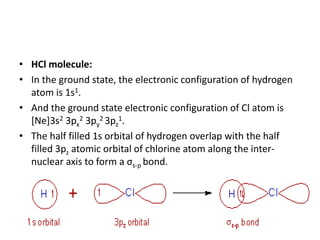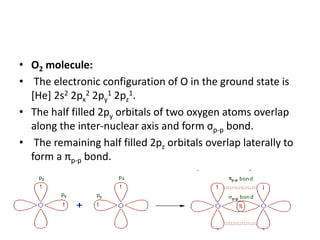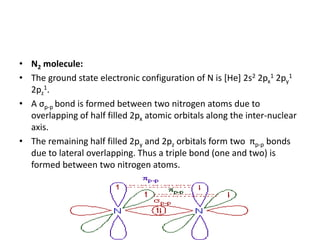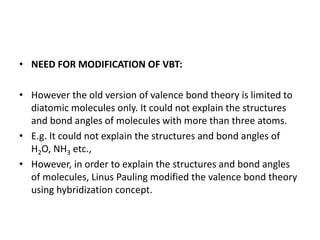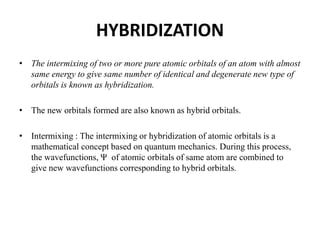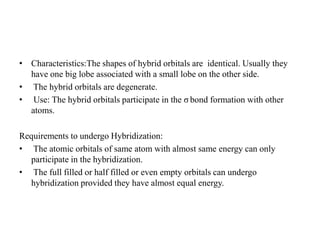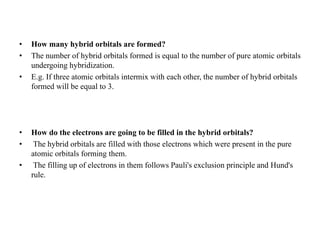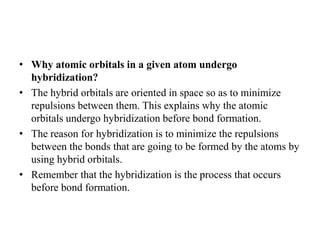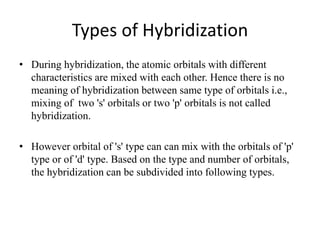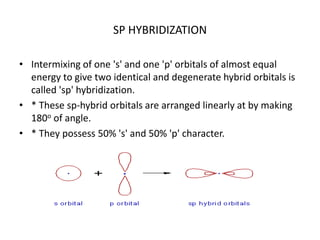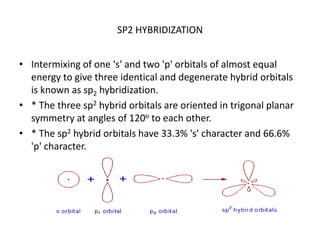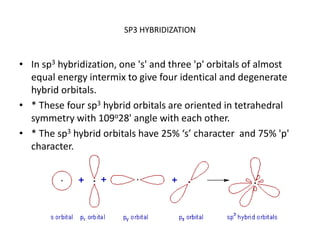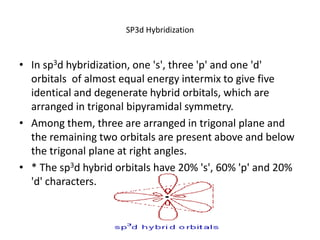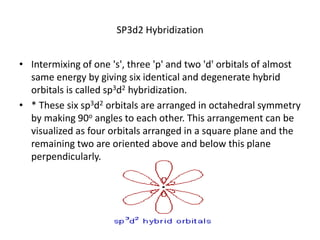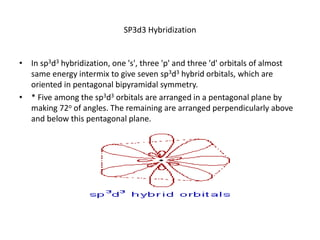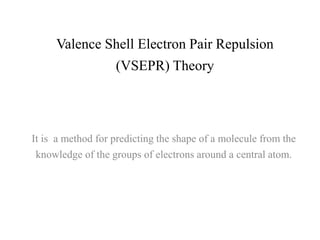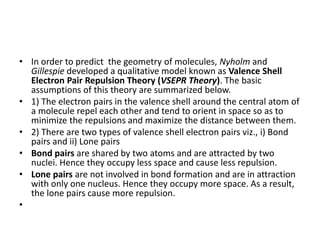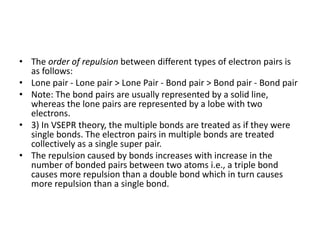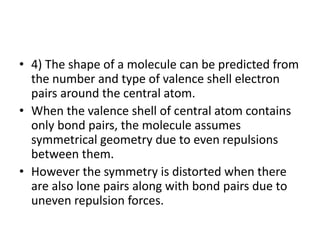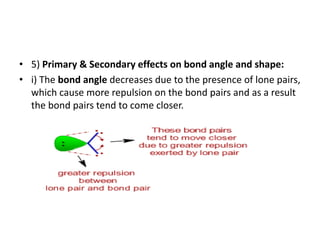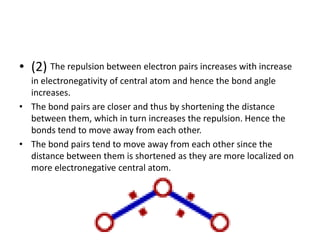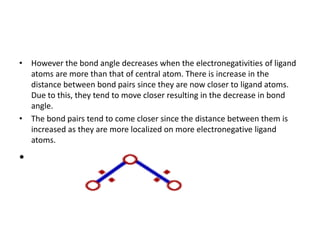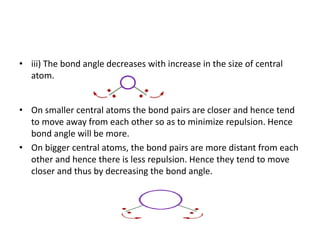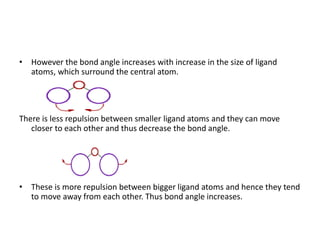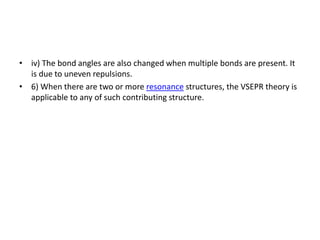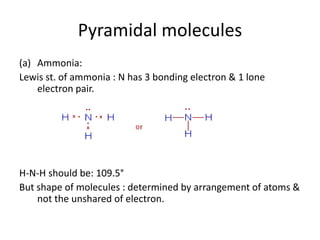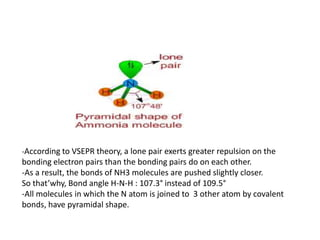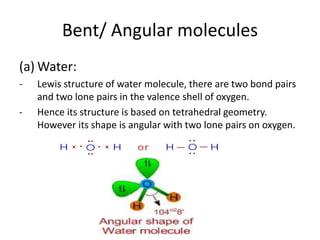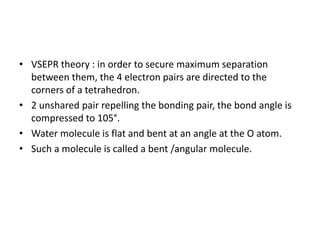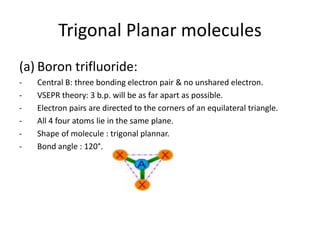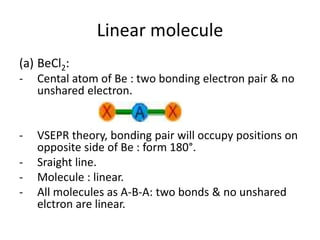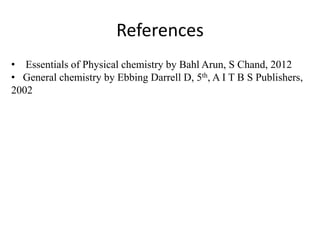1 de 49
Anúncio

### B.sc(microbiology and biotechnology and biochemistry) ii inorganic chemistry unit 3.2 covalent bond

1. Course: B.Sc. Microbiology/Biotechnology/Biochemistry Sem II Sub: Inorganic Chemistry Unit 3.2 Chemical Bonding
2. Covalent bond A link between atoms due to the sharing of two electrons. This bond forms between atoms of two non metallic elements. • Element : A substance that cannot be chemically converted into simpler substances; a substance in which all of the atoms have the same number of protons and therefore the same chemical characteristics. • Compound : A substance that contains two or more elements, the atoms of these elements always combining in the same whole- number ratio. • Mixture : A sample of matter that contains two or more pure substances (elements and compounds) and has variable composition.
3. • H,O,Br,F,I,N,Cl elements are all covalently bonded. • H2, O2, Br2, F2, I2, N2, Cl2. • Non polar covalent bond: • If the electrons are shared equally, there is a even distribution of the negative charge for the electrons in the bond, so there is no partial charges on the atoms. The bond is called a nonpolar covalent bond. • e.g.,BF3, O2, CH4
4. Polar covalent bond: • If one atom in the bond attracts electrons more than the other atom, the electron negative charge shifts to that atom giving it a partial negative charge. The other atom loses negative charge giving it a partial positive charge. The bond is called a polar covalent bond. • e.g.,HF, NH3, O3.
5. Naming simple molecules • Must memorize the prefixes • RULES: if there is only one of the first atom than don’t use a prefix, otherwise use a prefix. • Ex: CO = carbon monoxide • Ex: P2O4 = diphosphorous tetroxide Prefix Number Mono 1 Di 2 Tri 3 Tetra 4 Penta 5 Hexa 6 Hepta 7 Octa 8 Nona 9 Deca 10
6. Overlap of Atomic Orbitals The sharing of electrons between atoms is viewed as an overlap of atomic orbitals of the bonding atoms.
7. When H – H distance = 74 pm, Repulsion = Attraction  strongest bond  optimal overlap  lowest energy 74 pm
8. At H – H distance > 74 pm, Repulsion < Attraction  weaker bond  too little overlap  atoms come closer 74 pm > 74 pm
9. At H – H distance < 74 pm, Repulsion > Attraction  weaker bond  too much overlap  atoms get further apart 74 pm < 74 pm
10. Valence bond theory • The valence bond theory was proposed by Heitler and London to explain the formation of covalent bond quantitatively using quantum mechanics. Later on, Linus Pauling improved this theory by introducing the concept of hybridization. • The main postulates of this theory are as follows: • * A covalent bond is formed by the overlapping of two half filled valence atomic orbitals of two different atoms. • * The electrons in the overlapping orbitals get paired and confined between the nuclei of two atoms. • * The electron density between two bonded atoms increases due to overlapping. This confers stability to the molecule. • * Greater the extent of overlapping, stronger is the bond formed. • * The direction of the covalent bond is along the region of overlapping of the atomic orbitals i.e., covalent bond is directional. • * There are two types of covalent bonds based on the pattern of overlapping as follows:
11. • i) σ-bond: The covalent bond formed due to overlapping of atomic orbital along the inter nucleus axis is called σ-bond. It is a stronger bond and cylindrically symmetrical. • Depending on the types of orbitals overlapping, the σ-bond is divided into following types: • σs-s bond: •
12. σp-p bond: σs-p bond:
13. π-bond: The covalent bond formed by sidewise overlapping of atomic orbitals is called π- bond. In this bond, the electron density is present above and below the inter nuclear axis. It is relatively a weaker bond since the electrons are not strongly attracted by the nuclei of bonding atoms.
14. illustration • H2 molecule: • The electronic configuration of hydrogen atom in the ground state is 1s1. • In the formation of hydrogen molecule, two half filled 1s orbitals of hydrogen atoms overlap along the inter-nuclear axis and thus by forming a σs-s bond.
15. • Cl2 molecule: • * The electronic configuration of Cl atom in the ground state is [Ne]3s2 3px 2 3py 2 3pz 1. • * The two half filled 3pz atomic orbitals of two chlorine atoms overlap along the inter-nuclear axis and thus by forming a σp-p bond.
16. • HCl molecule: • In the ground state, the electronic configuration of hydrogen atom is 1s1. • And the ground state electronic configuration of Cl atom is [Ne]3s2 3px 2 3py 2 3pz 1. • The half filled 1s orbital of hydrogen overlap with the half filled 3pz atomic orbital of chlorine atom along the inter- nuclear axis to form a σs-p bond.
17. • O2 molecule: • The electronic configuration of O in the ground state is [He] 2s2 2px 2 2py 1 2pz 1. • The half filled 2py orbitals of two oxygen atoms overlap along the inter-nuclear axis and form σp-p bond. • The remaining half filled 2pz orbitals overlap laterally to form a πp-p bond. • * Thus a double bond (one σp-p and one πp-p) is formed between two oxygen atoms.
18. • N2 molecule: • The ground state electronic configuration of N is [He] 2s2 2px 1 2py 1 2pz 1. • A σp-p bond is formed between two nitrogen atoms due to overlapping of half filled 2px atomic orbitals along the inter-nuclear axis. • The remaining half filled 2py and 2pz orbitals form two πp-p bonds due to lateral overlapping. Thus a triple bond (one and two) is formed between two nitrogen atoms.
19. • NEED FOR MODIFICATION OF VBT: • However the old version of valence bond theory is limited to diatomic molecules only. It could not explain the structures and bond angles of molecules with more than three atoms. • E.g. It could not explain the structures and bond angles of H2O, NH3 etc., • However, in order to explain the structures and bond angles of molecules, Linus Pauling modified the valence bond theory using hybridization concept.
20. HYBRIDIZATION • The intermixing of two or more pure atomic orbitals of an atom with almost same energy to give same number of identical and degenerate new type of orbitals is known as hybridization. • The new orbitals formed are also known as hybrid orbitals. • Intermixing : The intermixing or hybridization of atomic orbitals is a mathematical concept based on quantum mechanics. During this process, the wavefunctions, Ψ of atomic orbitals of same atom are combined to give new wavefunctions corresponding to hybrid orbitals.
21. • Characteristics:The shapes of hybrid orbitals are identical. Usually they have one big lobe associated with a small lobe on the other side. • The hybrid orbitals are degenerate. • Use: The hybrid orbitals participate in the σ bond formation with other atoms. Requirements to undergo Hybridization: • The atomic orbitals of same atom with almost same energy can only participate in the hybridization. • The full filled or half filled or even empty orbitals can undergo hybridization provided they have almost equal energy.
22. • How many hybrid orbitals are formed? • The number of hybrid orbitals formed is equal to the number of pure atomic orbitals undergoing hybridization. • E.g. If three atomic orbitals intermix with each other, the number of hybrid orbitals formed will be equal to 3. • How do the electrons are going to be filled in the hybrid orbitals? • The hybrid orbitals are filled with those electrons which were present in the pure atomic orbitals forming them. • The filling up of electrons in them follows Pauli's exclusion principle and Hund's rule.
23. • Why atomic orbitals in a given atom undergo hybridization? • The hybrid orbitals are oriented in space so as to minimize repulsions between them. This explains why the atomic orbitals undergo hybridization before bond formation. • The reason for hybridization is to minimize the repulsions between the bonds that are going to be formed by the atoms by using hybrid orbitals. • Remember that the hybridization is the process that occurs before bond formation.
24. Types of Hybridization • During hybridization, the atomic orbitals with different characteristics are mixed with each other. Hence there is no meaning of hybridization between same type of orbitals i.e., mixing of two 's' orbitals or two 'p' orbitals is not called hybridization. • However orbital of 's' type can can mix with the orbitals of 'p' type or of 'd' type. Based on the type and number of orbitals, the hybridization can be subdivided into following types.
25. SP HYBRIDIZATION • Intermixing of one 's' and one 'p' orbitals of almost equal energy to give two identical and degenerate hybrid orbitals is called 'sp' hybridization. • * These sp-hybrid orbitals are arranged linearly at by making 180o of angle. • * They possess 50% 's' and 50% 'p' character.
26. SP2 HYBRIDIZATION • Intermixing of one 's' and two 'p' orbitals of almost equal energy to give three identical and degenerate hybrid orbitals is known as sp2 hybridization. • * The three sp2 hybrid orbitals are oriented in trigonal planar symmetry at angles of 120o to each other. • * The sp2 hybrid orbitals have 33.3% 's' character and 66.6% 'p' character.
27. SP3 HYBRIDIZATION • In sp3 hybridization, one 's' and three 'p' orbitals of almost equal energy intermix to give four identical and degenerate hybrid orbitals. • * These four sp3 hybrid orbitals are oriented in tetrahedral symmetry with 109o28' angle with each other. • * The sp3 hybrid orbitals have 25% ‘s’ character and 75% 'p' character.
28. SP3d Hybridization • In sp3d hybridization, one 's', three 'p' and one 'd' orbitals of almost equal energy intermix to give five identical and degenerate hybrid orbitals, which are arranged in trigonal bipyramidal symmetry. • Among them, three are arranged in trigonal plane and the remaining two orbitals are present above and below the trigonal plane at right angles. • * The sp3d hybrid orbitals have 20% 's', 60% 'p' and 20% 'd' characters.
29. SP3d2 Hybridization • Intermixing of one 's', three 'p' and two 'd' orbitals of almost same energy by giving six identical and degenerate hybrid orbitals is called sp3d2 hybridization. • * These six sp3d2 orbitals are arranged in octahedral symmetry by making 90o angles to each other. This arrangement can be visualized as four orbitals arranged in a square plane and the remaining two are oriented above and below this plane perpendicularly.
30. SP3d3 Hybridization • In sp3d3 hybridization, one 's', three 'p' and three 'd' orbitals of almost same energy intermix to give seven sp3d3 hybrid orbitals, which are oriented in pentagonal bipyramidal symmetry. • * Five among the sp3d3 orbitals are arranged in a pentagonal plane by making 72o of angles. The remaining are arranged perpendicularly above and below this pentagonal plane.
31. Valence Shell Electron Pair Repulsion (VSEPR) Theory It is a method for predicting the shape of a molecule from the knowledge of the groups of electrons around a central atom.
32. • In order to predict the geometry of molecules, Nyholm and Gillespie developed a qualitative model known as Valence Shell Electron Pair Repulsion Theory (VSEPR Theory). The basic assumptions of this theory are summarized below. • 1) The electron pairs in the valence shell around the central atom of a molecule repel each other and tend to orient in space so as to minimize the repulsions and maximize the distance between them. • 2) There are two types of valence shell electron pairs viz., i) Bond pairs and ii) Lone pairs • Bond pairs are shared by two atoms and are attracted by two nuclei. Hence they occupy less space and cause less repulsion. • Lone pairs are not involved in bond formation and are in attraction with only one nucleus. Hence they occupy more space. As a result, the lone pairs cause more repulsion. •
33. • The order of repulsion between different types of electron pairs is as follows: • Lone pair - Lone pair > Lone Pair - Bond pair > Bond pair - Bond pair • Note: The bond pairs are usually represented by a solid line, whereas the lone pairs are represented by a lobe with two electrons. • 3) In VSEPR theory, the multiple bonds are treated as if they were single bonds. The electron pairs in multiple bonds are treated collectively as a single super pair. • The repulsion caused by bonds increases with increase in the number of bonded pairs between two atoms i.e., a triple bond causes more repulsion than a double bond which in turn causes more repulsion than a single bond.
34. • 4) The shape of a molecule can be predicted from the number and type of valence shell electron pairs around the central atom. • When the valence shell of central atom contains only bond pairs, the molecule assumes symmetrical geometry due to even repulsions between them. • However the symmetry is distorted when there are also lone pairs along with bond pairs due to uneven repulsion forces.
35. • 5) Primary & Secondary effects on bond angle and shape: • i) The bond angle decreases due to the presence of lone pairs, which cause more repulsion on the bond pairs and as a result the bond pairs tend to come closer.
36. • (2) The repulsion between electron pairs increases with increase in electronegativity of central atom and hence the bond angle increases. • The bond pairs are closer and thus by shortening the distance between them, which in turn increases the repulsion. Hence the bonds tend to move away from each other. • The bond pairs tend to move away from each other since the distance between them is shortened as they are more localized on more electronegative central atom.
37. • However the bond angle decreases when the electronegativities of ligand atoms are more than that of central atom. There is increase in the distance between bond pairs since they are now closer to ligand atoms. Due to this, they tend to move closer resulting in the decrease in bond angle. • The bond pairs tend to come closer since the distance between them is increased as they are more localized on more electronegative ligand atoms. •
38. • iii) The bond angle decreases with increase in the size of central atom. • On smaller central atoms the bond pairs are closer and hence tend to move away from each other so as to minimize repulsion. Hence bond angle will be more. • On bigger central atoms, the bond pairs are more distant from each other and hence there is less repulsion. Hence they tend to move closer and thus by decreasing the bond angle.
39. • However the bond angle increases with increase in the size of ligand atoms, which surround the central atom. There is less repulsion between smaller ligand atoms and they can move closer to each other and thus decrease the bond angle. • These is more repulsion between bigger ligand atoms and hence they tend to move away from each other. Thus bond angle increases.
40. • iv) The bond angles are also changed when multiple bonds are present. It is due to uneven repulsions. • 6) When there are two or more resonance structures, the VSEPR theory is applicable to any of such contributing structure.
41. Pyramidal molecules (a) Ammonia: Lewis st. of ammonia : N has 3 bonding electron & 1 lone electron pair. H-N-H should be: 109.5° But shape of molecules : determined by arrangement of atoms & not the unshared of electron.
42. -According to VSEPR theory, a lone pair exerts greater repulsion on the bonding electron pairs than the bonding pairs do on each other. -As a result, the bonds of NH3 molecules are pushed slightly closer. So that’why, Bond angle H-N-H : 107.3° instead of 109.5° -All molecules in which the N atom is joined to 3 other atom by covalent bonds, have pyramidal shape.
43. Bent/ Angular molecules (a) Water: - Lewis structure of water molecule, there are two bond pairs and two lone pairs in the valence shell of oxygen. - Hence its structure is based on tetrahedral geometry. However its shape is angular with two lone pairs on oxygen.
44. • VSEPR theory : in order to secure maximum separation between them, the 4 electron pairs are directed to the corners of a tetrahedron. • 2 unshared pair repelling the bonding pair, the bond angle is compressed to 105°. • Water molecule is flat and bent at an angle at the O atom. • Such a molecule is called a bent /angular molecule.
45. Trigonal Planar molecules (a) Boron trifluoride: - Central B: three bonding electron pair & no unshared electron. - VSEPR theory: 3 b.p. will be as far apart as possible. - Electron pairs are directed to the corners of an equilateral triangle. - All 4 four atoms lie in the same plane. - Shape of molecule : trigonal plannar. - Bond angle : 120°.
46. Linear molecule (a) BeCl2: - Cental atom of Be : two bonding electron pair & no unshared electron. - VSEPR theory, bonding pair will occupy positions on opposite side of Be : form 180°. - Sraight line. - Molecule : linear. - All molecules as A-B-A: two bonds & no unshared elctron are linear.
47. References • Essentials of Physical chemistry by Bahl Arun, S Chand, 2012 • General chemistry by Ebbing Darrell D, 5th, A I T B S Publishers, 2002
Anúncio